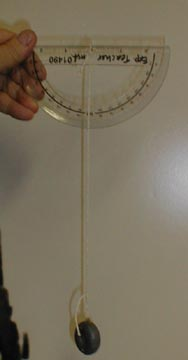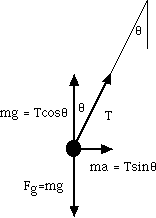Accelerometer

Give me a brakeA protractor can be used to measure angles and acceleration..

Introduction

You can use a protractor and a weight on a string to measure your horizontal acceleration.

Material

• a protractor
• a string about 25 cm or 10 inches long.
• a weight

Assembly

Tie the weight to the string.
Tie the other end of the string to the center hole of the protractor.

To Do and Notice

Face the direction of acceleration or deceleration.
Hold the flat top of the protractor level.
Hold the protractor in a vertical plane parallel to your direction of motion.

Accelerate or decelerate.

Measure the angular deflection of the string.

At zero acceleration the string hangs straight down, there is zero angular deflection.

The larger the angle the greater the acceleration.

What's Going On?

When the string is deflected by 45 degrees your acceleration is equal to 1g.

When the string is deflected by 60 degrees your acceleration is 2g.

The general equation for the acceleration, a, when the string is deflected by an angle, q, is
a = tan(
q).

 angle, q, degrees a, g's 14 0.25 27 0.5 45 1.0 64 2.0 72 3.0 76 4.0

So What?

The best cars with excellent tires can decelerate at 1 g.

They can go from 60 mi/hr (about 100 km/hr) to a stop in 3 seconds.

This also means that if you drive your car off a cliff it accelerates down at 1g and it will go 0 to 60 in 3 seconds. (0 to 100 km/hr in 3 seconds).

A top fuel dragster can accelerate at 4 g's, 0 to 60 mi/hr (10 km/hr) in under a second.

A truck hitting the gravel of a runaway truck ramp will decelerate at 3 g's

A car hitting a set of sand barrels, "the Fitch Barrier system" will decelerate at 5 g's.

Math Root

The force needed to accelerate the mass, m, at an acceleration, a, is F = ma.

Wow! an actual direct application of one of Newton's laws.When the bob hangs at an angle
q from the vertical,
the vertical component of the tension in the string cancels gravity
the horizontal component of the tension provides the acceleration.

This force is provided by the horizontal component of the Tension, T, in the string.

ma = T sin q

The vertical component of the tension in the string holds up the mass against the force of gravity , f = mg.

mg = T cos q

Find the ratio of ma/mg = a/g = T sin q / T cos q = tan q

Notice that the unknown tension and mass nicely vanishes!